# Java Programming Examples

Java programming is a language that is still dominating the Android world. There are almost a ton of famous Android applications written in Java. Some world-famous applications that use Java are Google, Amazon, LinkedIn, Uber, Spotify, etc.

I know, as a programmer, how to learn a language efficiently. We need to practice as much as possible. Therefore, I've written around 500 Java codes here, separated into more than 100 Java program articles.

Since there are a lot of programs, I've written them in Java. And of course, all those programs cannot be included in a single article. Therefore, I've divided those programs into separate articles.

This is basically the home page of Java programming examples. All the programs written here are well-tested and executed using or under one of the most famous Java IDEs, the Apache NetBeans IDE. This series of Java programming examples is designed to take care of beginners too. So that, in most of the article, I've first included the basic version of the code, then written the complete version of the same program.

And I assure you, after practicing all the programs written here, that you will be successful. You'll feel much better than ever before. Therefore, it becomes important to experiment with all the Java programs written here. As much as you practice, the more you build your skill in Java.

## Simple Java Programs for Beginners

Here is a list of some simple Java programs written for beginners: So if you're a beginner Java programmer, then you must practice these Java programs before visiting the other Java programs. These Java programs may boost your knowledge in the field of Java so that you can easily get started.

## List of the Top 100 Java Programs

Here is the list of the top 100 Java programs. The series of Java programs provided here is not limited to this list of Java programs. You'll get much more than this. But these are the common programs that are almost available in most of the projects and assignments.

Now, before starting the series of Java programming examples, let's first take a look at some of the basic Java programs along with their explanations.

## Java Program Example No. 1

The Java program written below is the very basic and simplest program that can be written in Java. Let's see the code and its output. Then its explanation, to get some idea about what is going behind the code written in Java.

```public class CodesCracker
{
public static void main(String[] args)
{
System.out.println("Hey, Java is Fun!");
}
}```

This program produces the output as shown in the snapshot given below: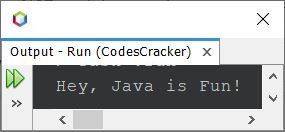In the above program, the following code:

`public class CodesCracker`

tells that a class named CodesCracker is defined using the keyword class. This class is declared public using the public keyword. Here, public is an access modifier. Declaring the class "public" means the class CodesCracker is accessible to all. And the following code:

`public static void main(String[] args)`

refers to the definition of the main() method. Every Java program must have this method. The execution of the program starts within the main() method.

Before the method main(), the keyword void refers to the return type of the method. Declaring a method void as its return type means the method does not return any value. And of course, we did not want the main() method to return any value.

Using the method as a static type means that we do not need to create an object to invoke the method. And of course, the main() method is to be called without any object; therefore, it is defined using static. And the keyword public is again an access modifier.

And the String[] args indicate an array of a sequence of strings that are passed to the method main(). This happens when the program is executed. For example, if you execute the Java program using or via the command line:

`java CodesCracker Hey, Java is Fun!`

Then, the array will store: ["Hey,", "Java", "is", "Fun!"].

And finally, inside the main() function, the code System.out.println() is used to print content on the output screen. For example, in the above program, I've passed the string "Hey, Java is Fun!". Therefore, this string gets printed on the output screen.

The above program can also be written as:

```public class CodesCracker
{
public static void main(String[] args)
{
String str = "Hey, Java is Fun!";
System.out.println(str);
}
}```

You'll get the same output as the previous program's output.

Now, in short, if I say that every Java program contains a main() method inside the class with a name the same as the Java application's or program's source code name, that is:

```public class CodesCracker
{
public static void main(String[] args)
{
// your Java code goes here
}
}```

where CodesCracker is the name of the class. In your case, the only change that may occur is this name. Other things would be the same. And in the section // your Java code goes here, you'll write your Java code to do whatever you want. Now let's take a look at some more Java program examples, given below.

## Java Program Example No. 2

This is the second version of a program written in Java. This program uses a loop to execute a statement multiple times.

```public class CodesCracker
{
public static void main(String[] args)
{
for(int i=0; i<10; i++)
System.out.println(i);
}
}```

The output produced by this Java program will be: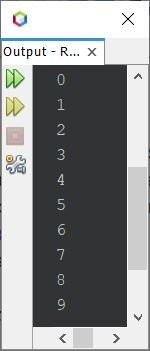As already told, the execution of the Java program starts with the main() method. Therefore, inside the main() method, there is a for loop defined. The for loop starts its execution in such a way that the first statement gets executed initially and only once. Therefore, using the first statement, that is:

`int i=0;`

a variable i gets defined, which is of the int type. And initialized with a value of 0. Now, while entering the body of the loop, every time the compiler checks the condition of the loop, That is, the second statement (condition-checking) gets executed.

`i<10;`

now the variable i gets replaced with its value. Since its value for now is 0, 0<10 evaluates to be true. Since the condition evaluates to be true, program flow goes inside the loop. Now inside the loop, the statement:

`System.out.println(i);`

gets executed. That prints the value of i on the output screen. Therefore, 0 gets printed on the output. When the statement of the loop gets executed, the compiler goes to the third statement of the loop, that is:

`i++`

The value of i gets incremented by 1. So i = 1 now. As already told, before entering the loop, the condition-checking statement (the second statement) gets evaluated. Since i<10 or 1<10 again evaluates to be true, program flow again goes inside the loop and prints the new value of i. That is, 1 this time. This process continues until the condition is evaluated as false.

## Java Program Example No. 3

Now let's create another program in Java that receives the name of a user and prints some string on the output, along with the entered name:

```import java.util.Scanner;

public class CodesCracker
{
public static void main(String[] args)
{
String name;
Scanner scan = new Scanner(System.in);

name = scan.nextLine();

System.out.println("\nHey,");
System.out.println(name);
System.out.println("Are you excited ?");
}
}```

The snapshot given below shows the sample run of the above Java program, with user input James as the name: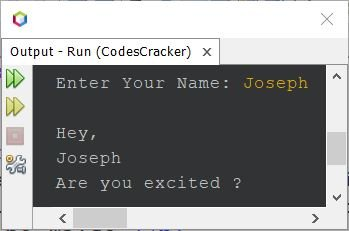Because in Java, to scan input from the user, we need to use the Scanner class. And the Scanner class is defined inside the java.util package. Therefore, before using the Scanner class to scan the input, I've imported the package and then defined an object scan of the Scanner class to scan input from the user. Don't worry, you'll learn everything, step by step, in this series of Java program examples.

In the above program, the \n used in the second System.out.println() statement is an escape sequence. That inserts a newline to the output screen so that the next output thing will get printed from the next line. Therefore, the string Hey gets printed one line after the user's input. Also from the above program, the last three System.out.println() statements can be written as:

`System.out.println("\nHey,\n" +name+ "\nAre you excited ?");`

That is, in the above statement:

`"\nHey,\n" +name+ "\nAre you excited ?"`

is composed of three strings; the first one is:

`"\nHey,\n"`

which is the string itself. And the second one is:

`name`

which is indirectly equal to "Joseph" entered by the user, according to the sample run given above. Since the entered string is stored in the name variable. Therefore, wherever you write a name inside the main() method, it evaluates to "Joseph". And the third one is:

`"\nAre you excited ?"`

which is itself a string. And using the + operator, I've added all these three strings. So the output will be the same as in the previous program's sample run.

## Java Program Example No. 4

This program does not receive any input from the user. This program only declares four variables of the int type. And initialized some values to the three variables, and the summation of all three variables' values gets initialized to the fourth variable. The value of the fourth variable gets printed on the output screen using the System.out.println() statement.

```public class CodesCracker
{
public static void main(String[] args)
{
int a, b, c, res;
a = 10;
b = 20;
c = 30;
res = a+b+c;
System.out.println(res);
}
}```

The output produced by the above Java program will be:

`60`

## Java Program Example No. 5

This is the same program as the previous one. The only difference is that the values of three variables are received by the user at run-time of the program:

```import java.util.Scanner;

public class CodesCracker
{
public static void main(String[] args)
{
Scanner s = new Scanner(System.in);
System.out.print("Enter Three Numbers: ");
int a = s.nextInt();
int b = s.nextInt();
int c = s.nextInt();
int res = a+b+c;
System.out.println("\nResult = " +res);
}
}```

The output produced by the above Java program, with user input of 10, 20, and 30 as three numbers, is shown in the snapshot given below: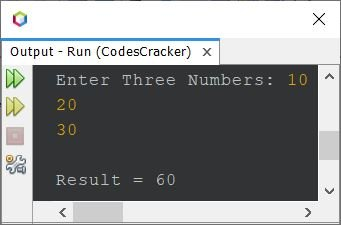## Java Program Example No. 6

Here is the same program as the previous one, except this program works with both int and float type values. That is, if the user enters a whole number or a real number, this program works for both.

```import java.util.Scanner;

public class CodesCracker
{
public static void main(String[] args)
{
Scanner s = new Scanner(System.in);
System.out.print("Enter Three Numbers: ");
float a = s.nextFloat();
float b = s.nextFloat();
float c = s.nextFloat();
float res = a+b+c;
System.out.println("\nResult = " +res);
}
}```

Here is its sample run with user input (10.43, 20.53, and 30.53):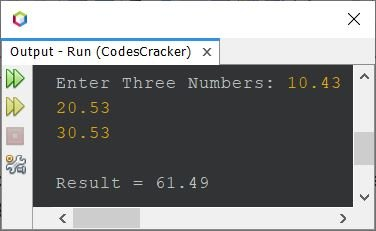## Java Program Example No. 7

Here is another program written in Java that receives two values of different types.

```import java.util.Scanner;

public class CodesCracker
{
public static void main(String[] args)
{
String name;
int n;
Scanner scan = new Scanner(System.in);

name = scan.nextLine();
System.out.print("Enter the Value of n: ");
n = scan.nextInt();

System.out.println("\nPrinting \"" +name+ "\", " +n+ " times.");
for(int i=0; i<n; i++)
System.out.println(name);
}
}```

The sample output produced by the above Java program with user input Mark as a name or string and 10 as the value of n is shown in the snapshot given below:The above seven Java program examples are written to show you the demo of how the program can be written in Java. From now on, one by one, you'll get much more programs written in Java in different ways.

#### Other Language Examples

Java Online Test

« Java Tutorial Next Program »